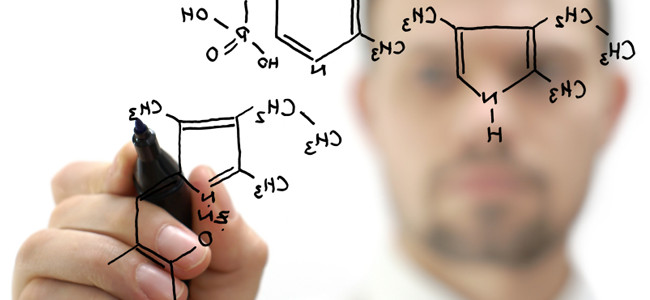Chapter 1 Test: Introduction To Chemistry

35 Questions | Total Attempts: 432Settings• 1.
Polymer chemists investigate the extremely long repeating chains of molecules known as polymers, also commonly known as
• A.

Strands

• B.

Plastics

• C.

• D.

Rubber

• 2.
Which one of the following is an example of a physical property?
• A.

Dynamite explodes

• B.

Meat rots if it is not refrigerated

• C.

Gasoline burns

• D.

Ice floats on top of liquid water

• E.

A silver platter tarnishes

• 3.
What is the relationship between the two substances shown below?
• A.

Identical substances

• B.

Isotopes

• C.

Isomers

• D.

Enantiomers

• 4.
How many bonds does nitrogen typically form?
• A.

1

• B.

2

• C.

3

• D.

4

• 5.
How many bonds does hydrogen typically form?
• A.

1

• B.

2

• C.

3

• D.

4

• 6.
How many bonds does oxygen typically form?
• A.

1

• B.

2

• C.

3

• D.

4

• 7.
How many bonds does carbon typically form?
• A.

1

• B.

2

• C.

3

• D.

4

• 8.
H-O-H is an example of a ________ formula
• A.

Molecular

• B.

Structural

• 9.
What is the relationship between the two substances shown below?
• A.

Isomers

• B.

Isotopes

• C.

Isotoners

• D.

They are identical

• 10.
Why is chemistry awesome?
• A.

Chemists get to blow stuff up

• B.

Chemistry is a gateway to great careers and fields of study

• C.

Chemistry can provide deep molecular answers to questions

• D.

All of the above

• 11.
What is chemistry?
• A.

The study of chemicals

• B.

The study of matter and how it changes

• C.

The art of mixing chemicals together and the determination of the products that form

• D.

A measure of the compatibility between two people

• 12.
• A.

They mix chemicals

• B.

They study matter and how it changes

• C.

They create new drugs

• D.

They analyze substances

• 13.
What is a chemical?
• A.

A pure form of matter, also known as a substance.

• B.

A dangerous form of matter

• C.

A toxic form of matter

• D.

A chemical is a mixture of elements

• 14.
Scientist use chemistry to provide ____________ answers to problems, which arguably provide the deepest answers to many problems.
• A.

Mathematical

• B.

Molecular

• C.

Subatomic

• D.

Long

• 15.
What is a substance?
• A.

A single chemical or a mixture of chemicals

• B.

Two or more atoms bonded together

• C.

Any small sample which has been chemically investigated

• D.

A pure form of matter

• 16.
The study of how matter changes generally refers to the __________study of matter
• A.

Physical

• B.

Chemical

• C.

Biological

• D.

Toxicological

• 17.
The field of natural products is a branch of chemistry which focuses on those substances that are not man-made, but rather exist in the natural world.
• A.

True

• B.

False

• 18.
What is matter?
• A.

Anything that has mass

• B.

Anything that has mass and takes up space

• C.

Anything that has mass or volume

• D.

As the word implies, matter is anything that is important

• 19.
Which of the folowing is not an example of matter?
• A.

A grain of sand

• B.

Air

• C.

Energy

• D.

A very small rock

• 20.
Which of the following is an example of matter?
• A.

Happiness

• B.

Energy

• C.

A very small rock

• D.

Running

• 21.
What do all chemists do?
• A.

They all smoke cigarettes

• B.

They study the elements of life

• C.

They mix chemicals

• D.

They study matter

• 22.
A chemist walks into his laboratory, and using a device known as a mass spectrometer determines the structure of a substance he is interested in.  He then performs medical tests on the substance to see if has potential as an experimental medicine. In chronological order, what two types of chemist has he behaved as?
• A.

Organic,, medicinal

• B.

Synthetic, polymer

• C.

Combinatorial, analytical

• D.

Analytical, medicinal

• 23.
Which fields are considered prerequisite to chemistry, meaning that they provide a foundation for studying chemistry, also meaning that they are a more basic, less applied field of study than chemistry?
• A.

Math and physics

• B.

Biology and the social sciences

• C.

Genetics and molecular orbital theory

• D.

Math and biology

• 24.
Watson and Crick determined that all living things have DNA molecules in each cell. Based on this, what types of chemists are Watson and Crick?
• A.

Biochemists

• B.

Analytical chemists

• C.

Medicinal chemists

• D.

Physical chemists

• 25.
A tentative explanation for a set of observations that can be tested by further experimentation is referred to as
• A.

Hypothesis

• B.

A law

• C.

A theory

• D.

None of the aboveBack to top